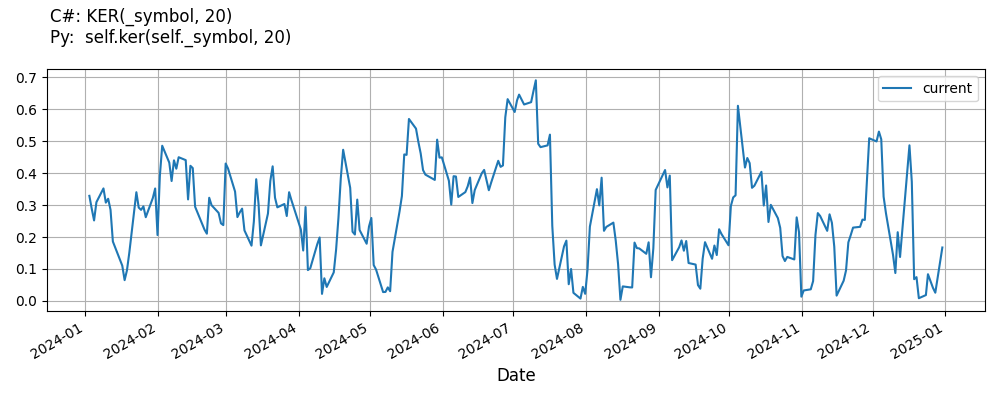# Supported Indicators

## Kaufman Efficiency Ratio

### Introduction

This indicator computes the Kaufman Efficiency Ratio (KER). The Kaufman Efficiency Ratio is calculated as explained here: https://www.marketvolume.com/technicalanalysis/efficiencyratio.asp

To view the implementation of this indicator, see the LEAN GitHub repository.

### Using KER Indicator

To create an automatic indicators for KaufmanEfficiencyRatio, call the KER helper method from the QCAlgorithm class. The KER method creates a KaufmanEfficiencyRatio object, hooks it up for automatic updates, and returns it so you can used it in your algorithm. In most cases, you should call the helper method in the Initialize method.

public class KaufmanEfficiencyRatioAlgorithm : QCAlgorithm
{
private Symbol _symbol;
private KaufmanEfficiencyRatio _ker;

public override void Initialize()
{
_ker = KER(_symbol, 20);
}

public override void OnData(Slice data)
{
{
// The current value of _ker is represented by itself (_ker)
// or _ker.Current.Value
Plot("KaufmanEfficiencyRatio", "ker", _ker);

}
}
}
class KaufmanEfficiencyRatioAlgorithm(QCAlgorithm):
def Initialize(self) -> None:
self.ker = self.KER(self.symbol, 20)

def OnData(self, slice: Slice) -> None:
# The current value of self.ker is represented by self.ker.Current.Value
self.Plot("KaufmanEfficiencyRatio", "ker", self.ker.Current.Value)



The following reference table describes the KER method:

### KER()1/1

            KaufmanEfficiencyRatio QuantConnect.Algorithm.QCAlgorithm.KER (
Symbol                           symbol,
*Int32                           period,
*Nullable<Resolution>      resolution,
*Func<IBaseData, Decimal>  selector
)


Creates an KaufmanEfficiencyRatio indicator for the symbol. The indicator will be automatically updated on the given resolution.

If you don't provide a resolution, it defaults to the security resolution. If you provide a resolution, it must be greater than or equal to the resolution of the security. For instance, if you subscribe to hourly data for a security, you should update its indicator with data that spans 1 hour or longer.

You can manually create a KaufmanEfficiencyRatio indicator, so it doesn't automatically update. Manual indicators let you update their values with any data you choose.

Updating your indicator manually enables you to control when the indicator is updated and what data you use to update it. To manually update the indicator, call the Update method with time/number pair, or an IndicatorDataPoint. The indicator will only be ready after you prime it with enough data.

public class KaufmanEfficiencyRatioAlgorithm : QCAlgorithm
{
private Symbol _symbol;
private KaufmanEfficiencyRatio _ker;

public override void Initialize()
{
_ker = new KaufmanEfficiencyRatio(20);
}

public override void OnData(Slice data)
{
if (data.Bars.TryGeValue(_symbol, out var bar))
{
_ker.Update(bar.EndTime, bar.Close);
}

{
// The current value of _ker is represented by itself (_ker)
// or _ker.Current.Value
Plot("KaufmanEfficiencyRatio", "ker", _ker);

}
}
}
class KaufmanEfficiencyRatioAlgorithm(QCAlgorithm):
def Initialize(self) -> None:
self.ker = KaufmanEfficiencyRatio(20)

def OnData(self, slice: Slice) -> None:
bar = slice.Bars.get(self.symbol)
if bar:
self.ker.Update(bar.EndTime, bar.Close)

# The current value of self.ker is represented by self.ker.Current.Value
self.Plot("KaufmanEfficiencyRatio", "ker", self.ker.Current.Value)



To register a manual indicator for automatic updates with the security data, call the RegisterIndicator method.

public class KaufmanEfficiencyRatioAlgorithm : QCAlgorithm
{
private Symbol _symbol;
private KaufmanEfficiencyRatio _ker;

public override void Initialize()
{
_ker = new KaufmanEfficiencyRatio(20);
RegisterIndicator(_symbol, _ker, Resolution.Daily);
}

public override void OnData(Slice data)
{
{
// The current value of _ker is represented by itself (_ker)
// or _ker.Current.Value
Plot("KaufmanEfficiencyRatio", "ker", _ker);

}
}
}
class KaufmanEfficiencyRatioAlgorithm(QCAlgorithm):
def Initialize(self) -> None:
self.ker = KaufmanEfficiencyRatio(20)
self.RegisterIndicator(self.symbol, self.ker, Resolution.Daily)

def OnData(self, slice: Slice) -> None:
# The current value of self.ker is represented by self.ker.Current.Value
self.Plot("KaufmanEfficiencyRatio", "ker", self.ker.Current.Value)



The following reference table describes the KaufmanEfficiencyRatio constructor:

### KaufmanEfficiencyRatio()1/2

            KaufmanEfficiencyRatio QuantConnect.Indicators.KaufmanEfficiencyRatio (
string  name,
int     period
)


Initializes a new instance of the KaufmanEfficiencyRatio class using the specified name and period.

### KaufmanEfficiencyRatio()2/2

            KaufmanEfficiencyRatio QuantConnect.Indicators.KaufmanEfficiencyRatio (
int  period
)


Initializes a new instance of the KaufmanEfficiencyRatio class using the specified period.

### Visualization

The following image shows plot values of selected properties of KaufmanEfficiencyRatio using the plotly library.You can also see our Videos. You can also get in touch with us via Discord.